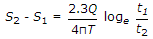# Civil Engineering - Water Supply Engineering

### Exercise :: Water Supply Engineering - Section 7

16.

Pick up the correct statement from the following :

 A. Due to boiling, the bacterias present in water can be destroyed B. Lime may be used for softening hard water C. Excess lime when added to water, raises the pH value of water D. the bacterias do not survive in water having pH value exceeding 9.5 E. All the above.

Explanation:

No answer description available for this question. Let us discuss.

17.

For the same draw down in two observations wells at distances r1 and r2, the times after start of pumping are t1 and t2 hours respectively. The relation which holds good is

 A.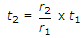B.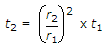C.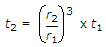D.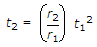E. none of these.

Explanation:

No answer description available for this question. Let us discuss.

18.

The valve fitted closely in a recess against an opening in a pipe, is generally

 A. wedge shaped circular disc B. spherical disc C. parallelopiped disc D. conical shaped circular disc.

Explanation:

No answer description available for this question. Let us discuss.

19.

For calculation of economical diameter D of a pipe in metres for a discharge Q to be pumped in cumecs, Lea suggested the empirical formula

 A. D = 0.22 Q B. D = 1.22 Q C. D = 2.22 Q D. D = 3.22 Q E. D = 1.33 Q.

Explanation:

No answer description available for this question. Let us discuss.

20.

S1 and S2 are the draw downs in an observation well at times t1 and t2 after pumping. For discharge Q and coefficient of transmissibility T, the relationship, is

 A.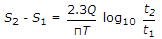B.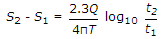C.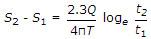D.